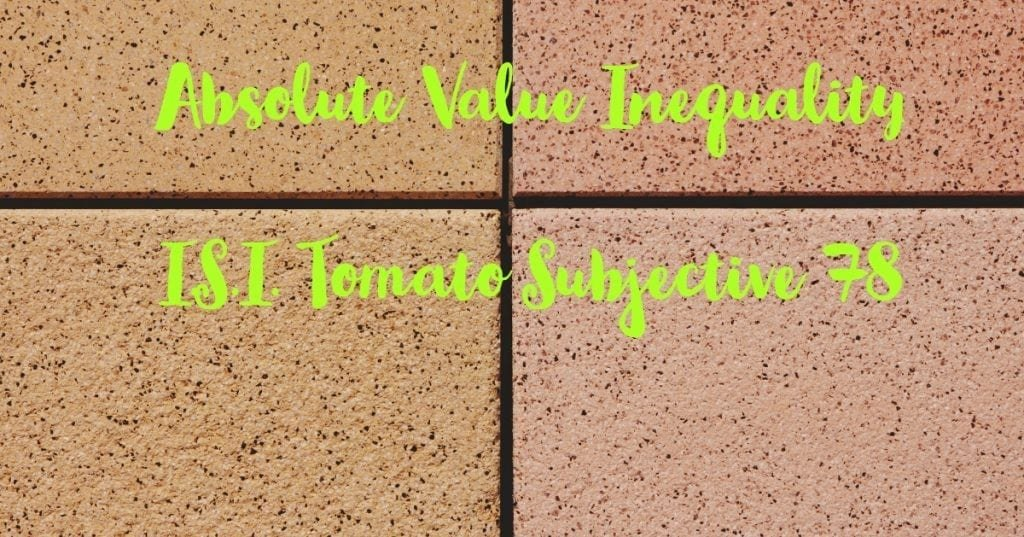How Cheenta works to ensure student success?
Explore the Back-Story

# Test of Mathematics Solution Subjective 78 -Absolute Value InequalityThis is a Test of Mathematics Solution Subjective 78 (from ISI Entrance). The book, Test of Mathematics at 10+2 Level is Published by East West Press. This problem book is indispensable for the preparation of I.S.I. B.Stat and B.Math Entrance.

Also visit: I.S.I. & C.M.I. Entrance Course of Cheenta

## Problem

For real numbers ${x}$, ${y}$ and ${\displaystyle{z}}$, show that
${\displaystyle{|x| + |y| + |z| {\le} |x + y - z| + |y + z - x| + |z + x - y|}}$.

## Solution

Applying Ravi transformation
${x = a + b}$, ${y = b + c}$ and ${z = c + a}$.
Our inequality reduces to ${\displaystyle{|a + b| + |b + c| + |c + a| {\le} |2b| + |2c| + |2a|}}$.
${\Leftrightarrow}$ ${\displaystyle{|a + b| + |b + c| + |c + a| {\le} 2(|a| + |b| + |c|)}}$.
Now we know, ${\displaystyle{|m + n| {\le} |m| + |n|}}$.
Applying this we get
L.H.S = ${\displaystyle{|a + b| + |b + c| + |c + a| {\le} |a| + |b| + |b| + |c| + |c| + |a|}}$
= ${\displaystyle{2(|a| + |b| + |c|)}}$
= R.H.S (proved)This is a Test of Mathematics Solution Subjective 78 (from ISI Entrance). The book, Test of Mathematics at 10+2 Level is Published by East West Press. This problem book is indispensable for the preparation of I.S.I. B.Stat and B.Math Entrance.

Also visit: I.S.I. & C.M.I. Entrance Course of Cheenta

## Problem

For real numbers ${x}$, ${y}$ and ${\displaystyle{z}}$, show that
${\displaystyle{|x| + |y| + |z| {\le} |x + y - z| + |y + z - x| + |z + x - y|}}$.

## Solution

Applying Ravi transformation
${x = a + b}$, ${y = b + c}$ and ${z = c + a}$.
Our inequality reduces to ${\displaystyle{|a + b| + |b + c| + |c + a| {\le} |2b| + |2c| + |2a|}}$.
${\Leftrightarrow}$ ${\displaystyle{|a + b| + |b + c| + |c + a| {\le} 2(|a| + |b| + |c|)}}$.
Now we know, ${\displaystyle{|m + n| {\le} |m| + |n|}}$.
Applying this we get
L.H.S = ${\displaystyle{|a + b| + |b + c| + |c + a| {\le} |a| + |b| + |b| + |c| + |c| + |a|}}$
= ${\displaystyle{2(|a| + |b| + |c|)}}$
= R.H.S (proved)

### Knowledge Partner